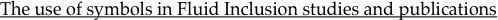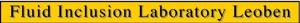Temperature measured with Microthermometry: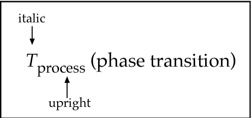Examples:
Melting of Ice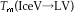where L = liquid, V = vapour, m = melting
abbreviated:
Tm(Ice)
Homogenization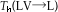where L = liquid, V = vapour, h = homogenization
abbreviated:
Th(total)
note: both "homogenization" and "melting" can be used
Composition:
Volume Fraction: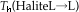where L = liquid, h = homogenization
abbreviated:
Tm(Halite)
end of file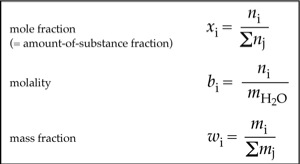note: parameter always in "italics", subscript always in "upright"
where mH2O is the mass of 55.51 mole H2O (= 1 kg)
Examples:
In a binary mixture of NaCl and H2O
xNaCl = 0.1
bNaCl = 6.1677
wNaCl = 26.5%
this corresponds to:
xNa+ = 0.0909
xCl- = 0.0909
xH2O = 0.8182
bNa+ = 6.1669
bCl- = 6.1669
wNa+ = 12.42%
wCl- = 17.94%
note: dissolved NaCl is dissociated in Na+ and Cl- ions
the system now includes three components (ternary): H2O , Na+ and Cl-
or
or
conformable to SI
Example: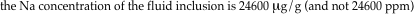where Vm is the molar volume, i is a phase (not a component!)
Standard International (S.I.)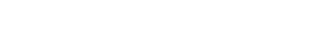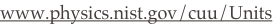Diamond LW (2003) Glossary: Terms and Symbols used in Fluid Inclusion Studies, in: Fluid Inclusions, Analysis and Interpretation (ed. Samson I, Anderson A, Marshall D), Short Course 32, Mineralogical Association of Canada, 365-374.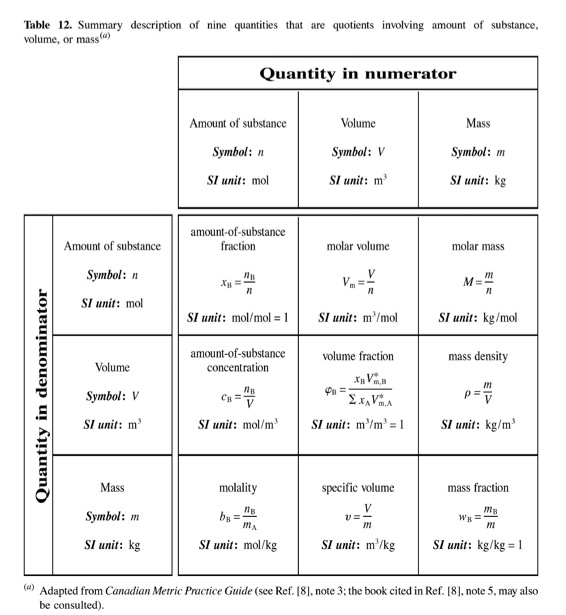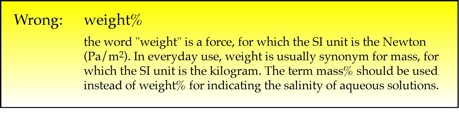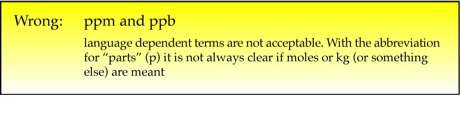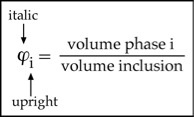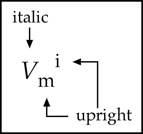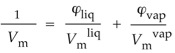Example:
Bakker RJ, Diamond LW (2006) Estimation of volume fractions of liquid and vapor phases in fluid inclusions, and definition of inclusion shapes. American Mineralogist, v.91, 635-657.
Volume:
Molar Volume (cm3/mol)Density (g/cm3)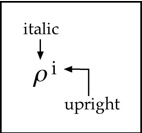note: in many drawing software, "symbols" (or "greek") cannot be put in italics, use a horizontal skew angle.
the volume fraction of the vapour phase is 30% (or 0.3)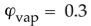Bulk molar volume of fluid inclusion:
Bulk density of fluid inclusion: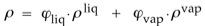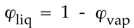In two phase fluid inclusions (LV):Pressure: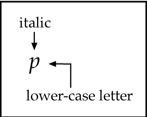The symbol for pressure is Pa (Pascal), or MPa, GPa. 1 Pa = 1 N/m2
The symbol for temperature is K (Kelvin), the use of degree Celsius is also allowed.
Do not use: bar (bar), standard atmosphere (atm), torr (torr), pound-force per square inch (psi)
Do not use: degree Fahrenheit, degree Rankine
Bakker RJ (2011) The use of quantities, units and symbols in fluid inclusion research. Berichte der Geologischen Bundesanstalt, nr. 87, 5-11. (pdf file)
Reference: